# Fight San Francisco Crime with fast.ai and Deepnote

UncategorizedDeepnoteposted by ODSC Community November 9, 2020

When most people picture San Francisco and the Bay Area, various positive connotations such as the Golden Gate Bridge, Chinatown, and...# Deepnote Setup & Integrations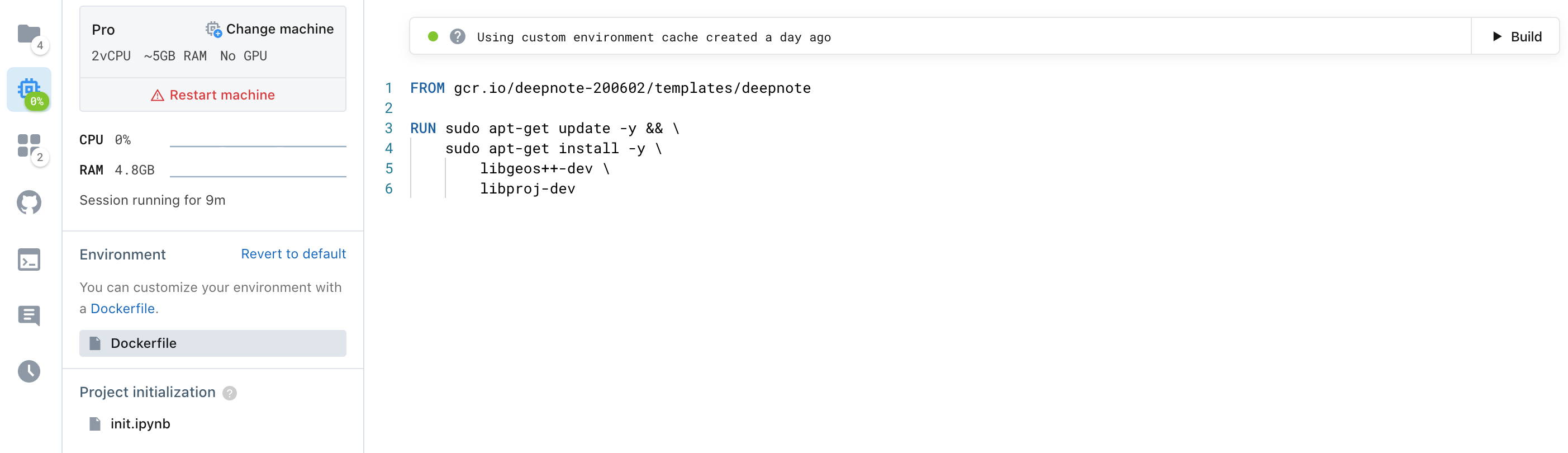A screenshot of the direct configuration of a Dockerfile in Deepnote [Image by Author]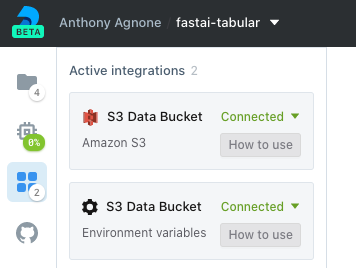A screenshot of the active integrations in the Deepnote notebook [Image by Author]
```import os
print(f"The contents of the S3 bucket connected to this notebook have been automatically transferred locally.\n"
f"S3 Bucket: s3://{os.environ['S3_BUCKET']}\n"
f"Local directory: /datasets/s3-data-bucket\n"
f"\nContents:")
!ls /datasets/s3-data-bucket```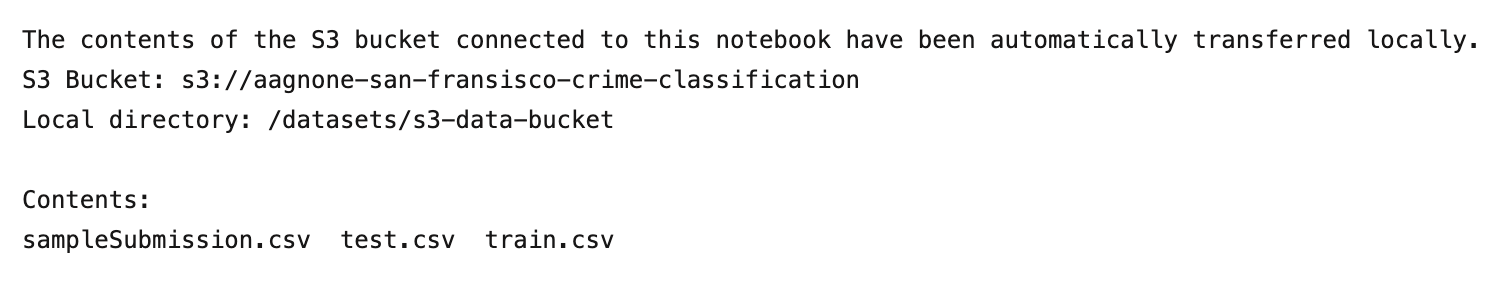The output of the code shown above [Image by author]

```random_state = 42
train.drop_duplicates(inplace=True)
train.reset_index(inplace=True, drop=True)
print(f"Loaded the dataset of {train.shape}-D features")

print(f"# train examples: {len(train)}\n# test examples: {len(test)}")
del test

# remove from clear outliers from the data set, allowing fast.ai to impute the values via `FillMissing` later on
train.replace({'X': -120.5, 'Y': 90.0}, np.NaN, inplace=True)```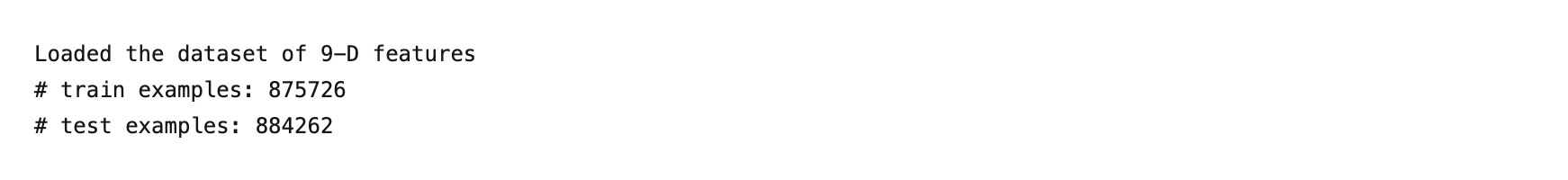The output of the code shown above [Image by author]

# Data Schema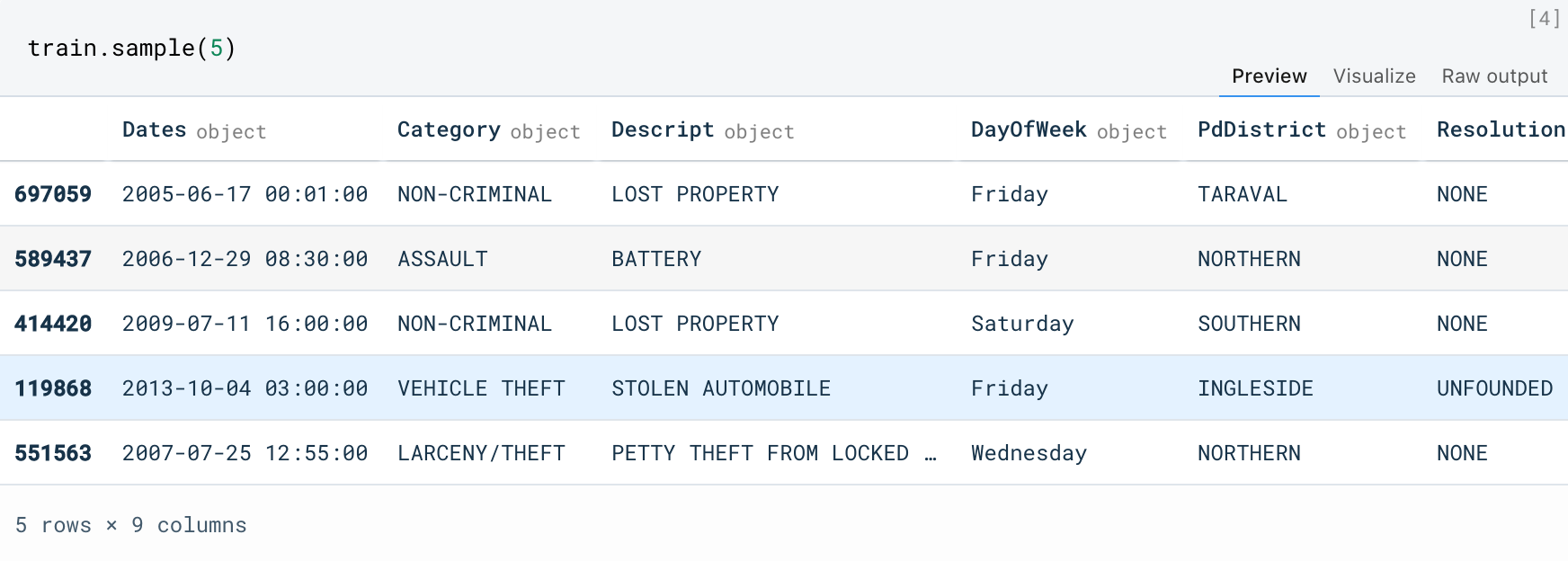A quick sample of the data set, prior to feature engineering [Image by author]

# Biases and Simplifications

```# drop unused columns
train.drop(["DayOfWeek", "Resolution", "Descript"], axis=1, inplace=True)

# target certain crime event categories
targeted_cats = [
'LARCENY/THEFT'
]
train["TargetedCategory"] = train.Category.isin(targeted_cats)
train.drop("Category", axis=1, inplace=True)
print(f"The {len(targeted_cats)} targeted categories occur in {100. * train.TargetedCategory.mean():.2f}% of the samples.")

```

```train['IsOnBlock'] = train['Address'].str.contains('block', case=False)

return re.sub(r"[0-9]+ [bB]lock of ", "", text)

def make_counts(values):
counts = Counter()
for value in values:
cur_counts = list(map(clean_road, value.split(" / ")))
counts.update(cur_counts)
return counts

# compute road counts, in preparation of the log road probability feature

# have a look at the most common roads in the data
plt.figure(figsize=(10, 10))
with sns.axes_style("whitegrid"):
ax = sns.barplot(
orient='h',
palette="Blues_r")

plt.xlabel('P(x)')

plt.show()```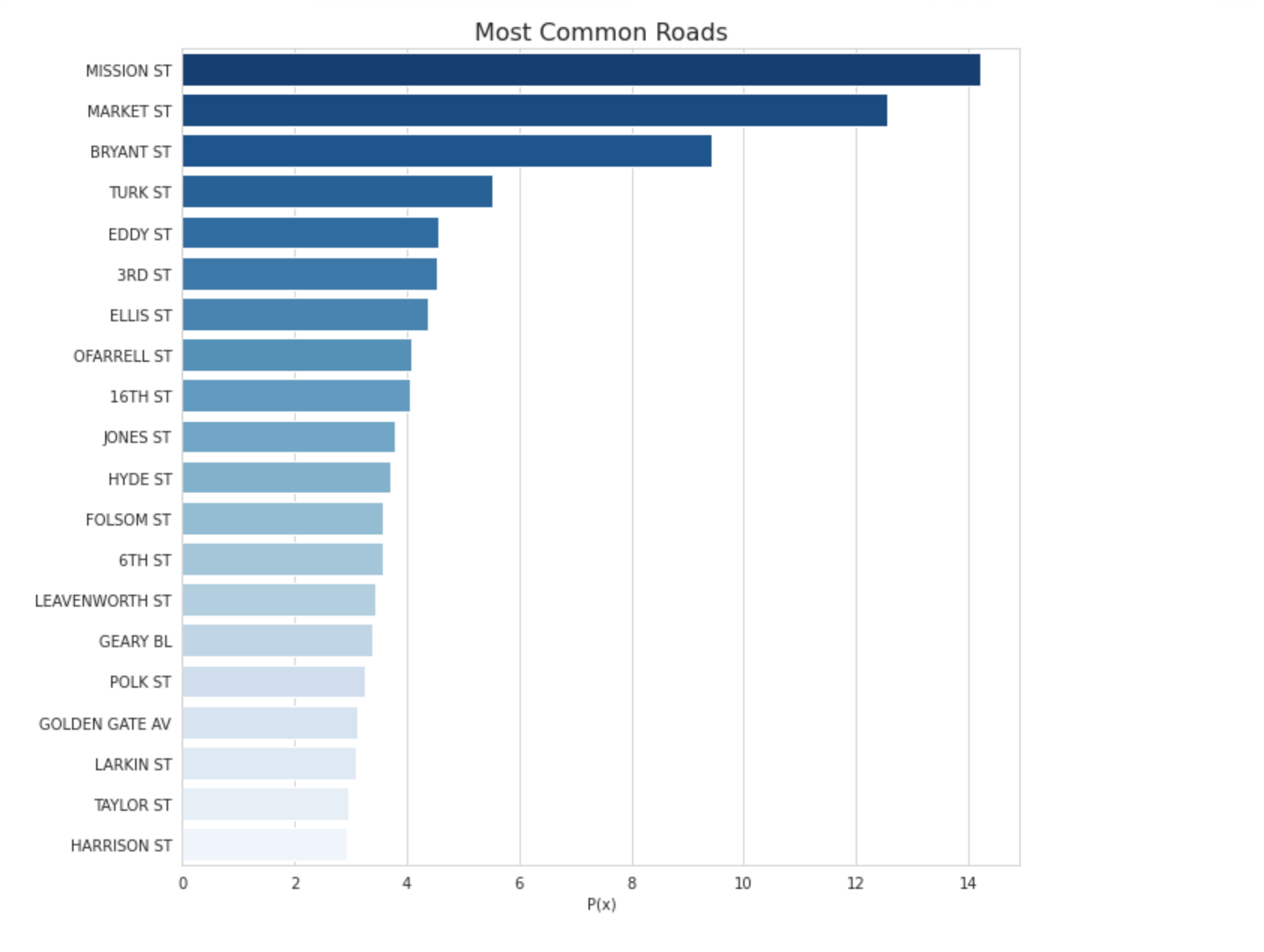The output of the code shown above [Image by author] # finalize the log road probability feature pd_counts = pd.Series(counts) log_probas = np.log(pd_counts / pd_counts.sum()) # have a look at the distribution of log road probabilities in the data plt.figure(figsize=(10, 10)) sns.displot(log_probas.values) plt.xlabel('ln(P(road))') plt.ylabel('P(x)') _ = plt.title("Distribution of Log Probas for Street Occurrence", fontdict={'fontsize': 16})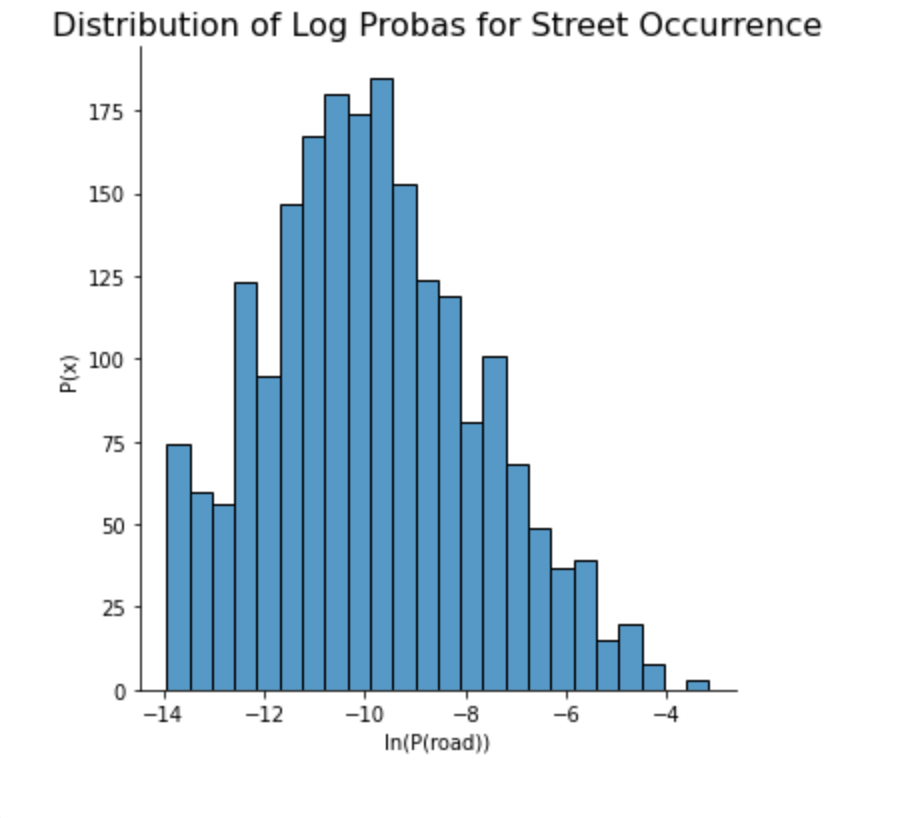The output of the code shown above [Image by author]
```# with the street probabilities, we can now assign them to each sample.
# as mentioned before, samples on street corners receive the mean of each street probability.
return np.mean([
])

```
```plt.figure(figsize=(12, 8))
plt.legend()
_ = plt.title("Label Separation for Log Road Probabilities")

```

# Data Set Profiling, Thanks to `pandas-profiling`

```profile = ProfileReport(train, minimal=True, title="SF Crime Data Set Profile")
profile.to_notebook_iframe()```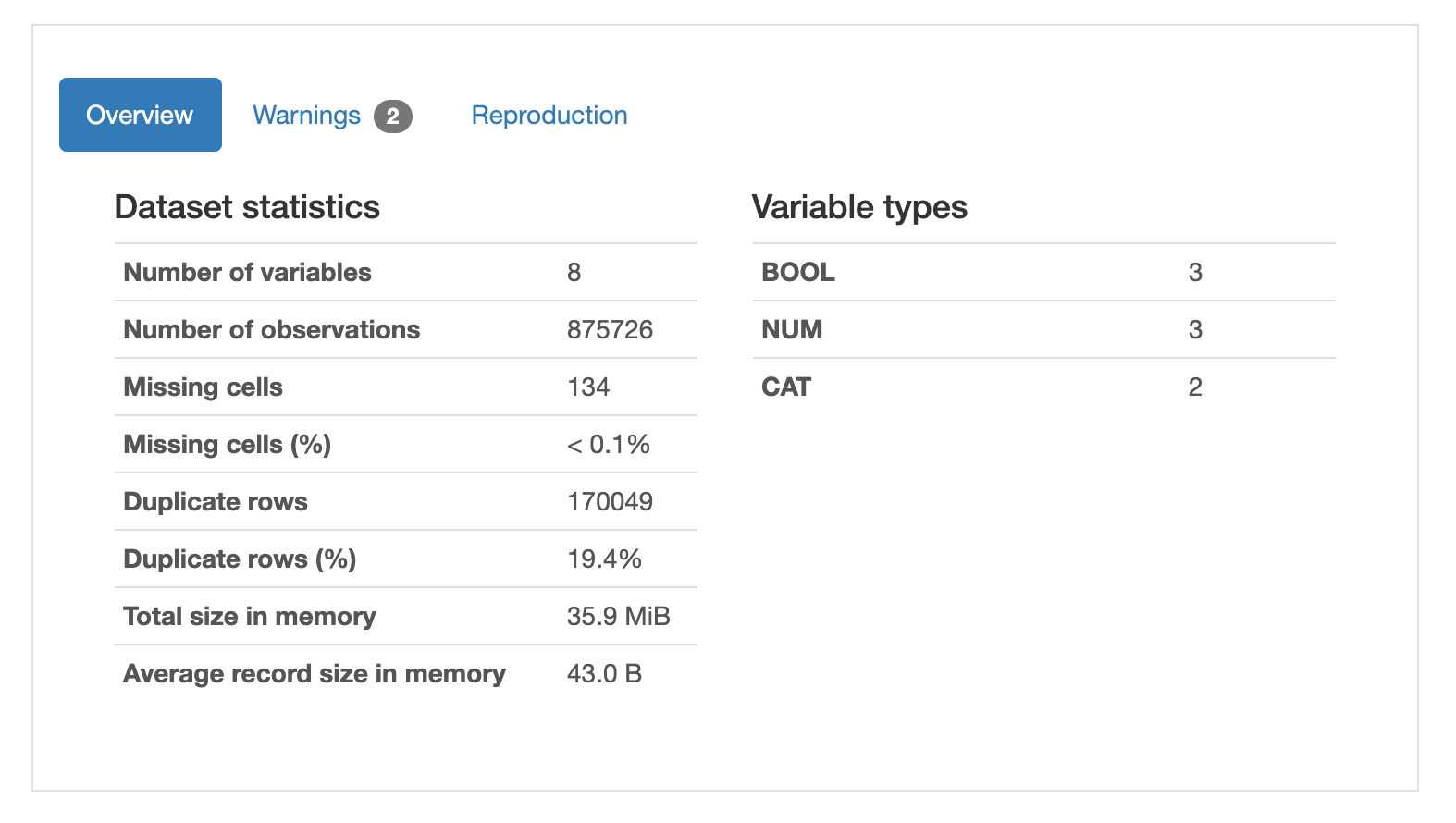Only the very beginning of a very detailed report from pandas_profiling [Image by author]

# Streamlined Data Preparation with `fast.ai`

```old_columns = train.columns
new_columns = list(set(train.columns) - set(old_columns))

print(f"add_datepart created {len(new_columns)} created new features")
for i, new_column in enumerate(new_columns):
print(f"  {i + 1}. {new_column}")```
```cont, cat = cont_cat_split(train, max_card=5, dep_var="TargetedCategory")
cat.remove("Dates")

print("Continuous columns:")
for i in range(0, len(cont), 4):
print('   ' + ', '.join(cont[i:i+4]))

print("Categorical columns:")
for i in range(0, len(cat), 4):
print('   ' + ', '.join(cat[i:i+4]))```

# Model Training

```def time_split(df, validation_pct=0.2):
df = df.sort_values("Dates")
split_date = df.loc[df.index[int(len(df) * (1 - validation_pct))], "Dates"]
return df.index[df["Dates"] <= split_date], df.index[df["Dates"] > split_date]

train_idx, validation_idx = time_split(train, validation_pct=0.2)
print(f"Training data has {len(train_idx)} samples from {train.loc[train_idx, 'Dates'].min()} to {train.loc[train_idx, 'Dates'].max()}")
print(f"Validation data has {len(validation_idx)} samples from {train.loc[validation_idx, 'Dates'].min()} to {train.loc[validation_idx, 'Dates'].max()}")

train.drop("Dates", axis=1, inplace=True)
to = TabularPandas(train,
procs=[Categorify, FillMissing, Normalize],
cat_names=cat,
cont_names=cont,
y_names="TargetedCategory",
splits=[list(train_idx), list(validation_idx)])
view rawsf_crime_12.py hosted with ❤ by GitHub```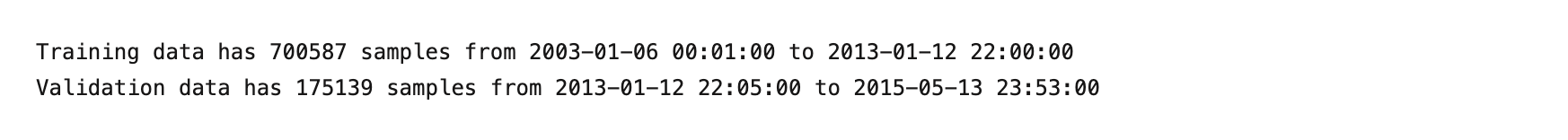The output of the code shown above [Image by author]

```X_train, y_train = to.train.xs, to.train.ys.values.ravel()
X_validation, y_validation = to.valid.xs, to.valid.ys.values.ravel()
print(f"The train set has {np.bincount(y_train)} positive labels.")
print(f"The validation set has {np.bincount(y_validation)} positive labels.")```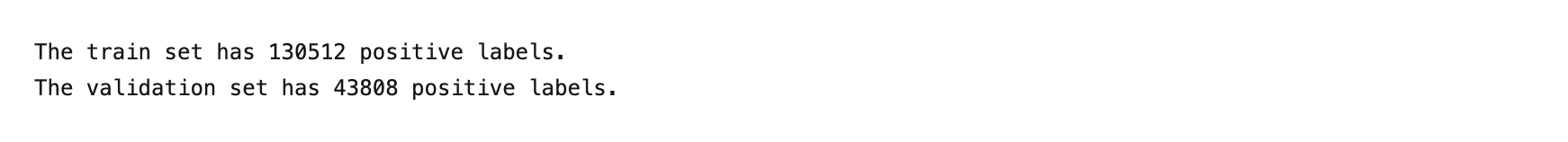The output of the code shown above [Image by author]

```params = {
'boosting': 'gbdt',
'objective': 'binary',
'is_unbalance': True,
'num_class': 1,
'learning_rate': 0.1,
}
train_data = lgb.Dataset(X_train, label=y_train, categorical_feature=['PdDistrict'])
model = lgb.train(params, train_data, 250)
y_train_pred = model.predict(X_train)
y_pred = model.predict(X_validation)

joblib.dump(model, "model.jbl")
print("Saved trained model")```

# Score Distributions

```fig = make_subplots(
rows=1, cols=2,
subplot_titles=(
"Classwise Score Distributions",
"Train vs Validation Score Distributions"
)
)

# class-wise score distributions
fig_distplots = ff.create_distplot(
["Negative", "Positive"],
show_hist=False, show_rug=False,
)
for trace in fig_distplots.select_traces():
fig.update_xaxes(range=(0, 1), row=1, col=1)
fig['layout']['xaxis2']['title'] = dict(text='Score')
fig['layout']['yaxis2']['title'] = dict(text='P(Score)')

# train vs validation score distributions
fig_distplots = ff.create_distplot(
[y_train_pred, y_pred],
["Training", "Validation"],
show_hist=False, show_rug=False
)
for trace in fig_distplots.select_traces():
fig.update_xaxes(range=(0, 1), row=1, col=2)
fig['layout']['xaxis2']['title'] = dict(text='Score')
fig['layout']['yaxis2']['title'] = dict(text='P(Score)')
fig.update_layout(showlegend=False)

fig.show()```

# Confusion Matrix

```c = confusion_matrix(y_validation, y_pred > 0.5, normalize='true')
fig = ff.create_annotated_heatmap(
c,
x=['Not Target', 'Target'],
y=['Not Target', 'Target'],
colorscale="Greens"
)
fig.update_xaxes(side="top", title="Prediction")
fig.update_yaxes(title="Truth")
fig.show()```

# Tradeoff Curves: ROC and Precision-Recall

```fpr, tpr, thresholds = roc_curve(y_validation, y_pred)

fig = make_subplots(
rows=1, cols=2,
subplot_titles=(
"ROC Curve",
"Precision vs Recall Curve"
)
)

# ROC curve
# add dotted line to show the performance of randomly guessing (50%)
x=[0, 1],
y=[0, 1],
line=dict(
color='royalblue',
width=2,
dash='dash'
)
), row=1, col=1)
fig.update_layout(showlegend=False)

# plot ROC curve, filling the margin above (or below!) the random guess line
x=fpr,
y=tpr,
fill='tonexty',
mode='lines',
), row=1, col=1)
fig['layout']['xaxis']['title'] = dict(text='FPR')
fig['layout']['yaxis']['title'] = dict(text='TPR')

# precision-recall curve
precision, recall, thresholds = precision_recall_curve(y_validation, y_pred)

fig_prc = px.area(
x=recall, y=precision,
title=f'Precision-Recall Curve (AUC={auc(fpr, tpr):.4f})',
labels=dict(x='Recall', y='Precision'),
width=700, height=500
)
fig['layout']['xaxis2']['title'] = dict(text='Recall')
fig['layout']['yaxis2']['title'] = dict(text='Precision')

fig.show()```

# Resources

Launch my Deepnote notebook directly
Kaggle competition for the SF crime data set
– A fantastic Kaggle notebook on the data set by Yannis Pappas (some code re-used here)
– San Francisco government’s OpenData initiative
fast.ai and, in particular here, the Tabular package.
– The `pandas_profiling` Github page.
– Plotly’s wonderful Python support.
– More from yours truly at Life With DataTwitter, and Medium

Originally posted here.## ODSC Community

The Open Data Science community is passionate and diverse, and we always welcome contributions from data science professionals! All of the articles under this profile are from our community, with individual authors mentioned in the text itself.

1